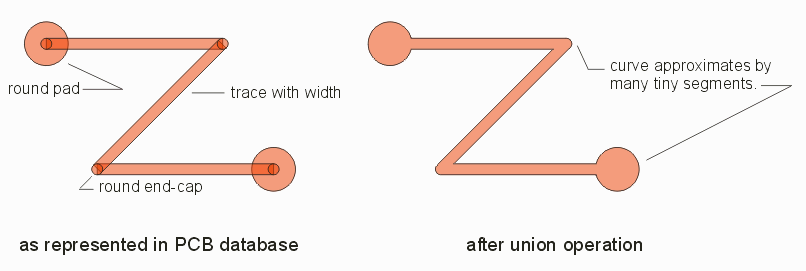### Introduction

Many databases make efficient user of composite curves - a region defined by a combination of arcs and segments. When such regions are run through a Boolean operation such as union, XOR or minus, the first step is normally to break up the curves into many short segments since Boolean routines do not work directly on curve data.

The output of the Boolean operation is a polygon - however to approximate the original curved edges of the region, many small segments are now used. This greatly increases the size of the database. In particular, if one is then extruding the 2D polygon into 3D, one produces a 3D solid with a large number of faces. This high face count then can cause meshing problems for finite element analysis tools.

It would be very helpful, then, to have a function that would take the output from a Boolean function, recognize the "curved" sections and replace the series of segments with a true curve.

Circularize is such a function.

### Definitions and an Example

Let's use an example from the printed circuit board world. Much of a PCB's conductor pattern consists of round pads connected with traces that have a finite width and which are drawn using a round aperture.In the PCB database the representation is very compact. A round symbol for the pad is inserted twice and a trace is assigned a width and given 4 coordinates. If we need to convert this into a "clean" polygon using a Boolean, the polygon we end up with has the same "footprint" but it will have a very high vertex count because the arcs that defined the round pads and bends in the trace are now approximated using a large number of small segments.If we created the 2D polygon so that we could extrude it into 3D (as we do for our SolidWorks importer) we find that our 3D solid model has a lot of faces. This is not a good thing as it greatly slows down both importation and operation of the 3D modeling engine.

What we want is to somehow recover the arcs and store the polygon as a composite curve.

Composite Curve

A composite curve consists of a series of elements where each element is either a line segment or an arc. The endpoint of one element acts as the starting point of the following element. A well behaved composite curve does not contain any zero length segments nor any arc with zero or infinite radius.And, of course, if we are going to use the composite curve to define a simple region then the first and last point must coincide and the curve should not self intersect.

Next Page: Function Description